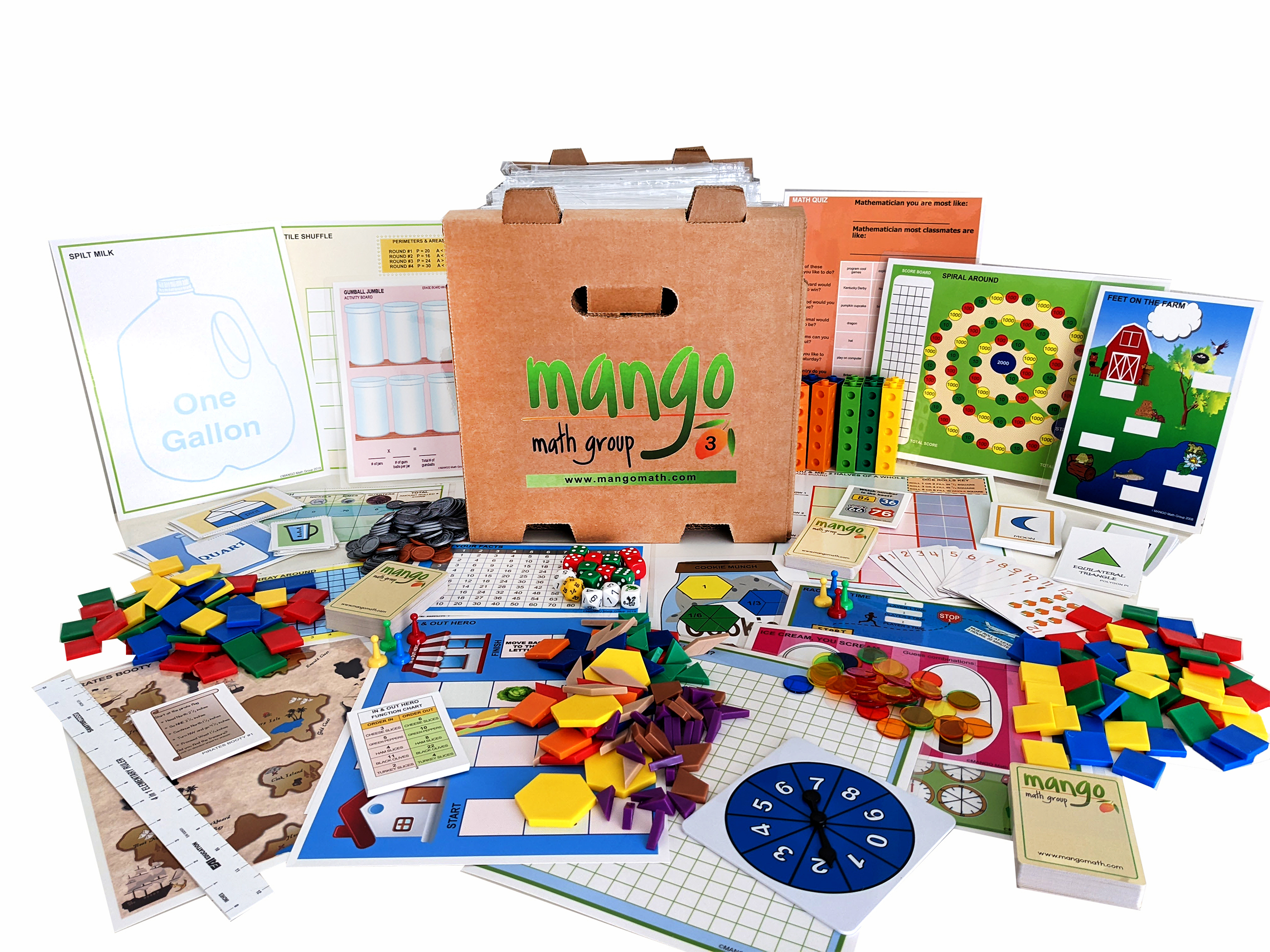#### Order Notice

As the economy opens back up, many of our suppliers are experiencing shortages of parts and raw materials, resulting in delays in shipments. As a result, we anticipate that it may take up to 2-4 weeks from the date we receive a purchase order for us to fulfill and ship any order for our products. We apologize for any inconvenience.

NEW!!

# Third Grade Deluxe Math Kit

Our Strong Evidence Based Third Grade Deluxe Math Kit has creative math games and activities for even more math fun! This contains 20 separate skill-building math lessons.

\$ 459.00 USD##### Product Details

MANGO Math's Third Grade Deluxe Math Kit has 20 separate skill building math lessons.

Each individual lesson offers visual and kinetic representation of math concepts that develop strong math knowledge, like:

• multiplication and division within 100
• fractions, especially unit fractions
• structure of rectangular arrays and area

MANGO Math's Strong Evidence Based Kits come with individually packaged, Grab-n-Go lessons that include instructions and reusable materials, pre-packaged in a resealable pouch. These are contained in an easy-to-carry crate that can be used year after year.

Grade-level math kits, set up in math center format, can meet the needs of 30+ students at a time. Lessons within a kit can be used by up to 4 students.

Every lesson is based on a game or activity that fosters curiosity, discussion and growth, through cooperation, collaboration and creative thinking.

Each MANGO Math grade level math kit is research-based and adheres to Common Core, TEKS and NCTM math standards.

Kits are great for use in regular classrooms, resource rooms, after school programs, STEM programs, libraries, math centers, summer camps, learning cooperatives, math clubs, community centers, and at home.

#### All the supplies below are included in this pack:

• 6-side dice
• 12-sided dice
• 20-sided dice
• Animal dice
• Bi-colored counters
• Transparent counters
• Stacking counters
• Play Money: pennies, nickels, dimes, quarters, half dollars, dollar bills, \$10 bill, \$100 bill
• Rulers
• Sand timer
• MANGO playing cards
• Fraction pattern blocks
• Game pawns
• Colored tiles
• Connecting cubes
• Fraction squares
• Plastic resealable pouches
• Easy to Follow direction
• Game boards
• Laminated writing boards
• Dry erase markers
• Curriculum Guide

## Third Grade Lessons with Standards

Got Room – Measurement: Recognize area as an attribute of plane figures and understand concepts of area measurement.

Pirates Booty – Measurement: Generate measurement data by measuring lengths using ruler marked with halves and fourths of an inch.

Race for Time – Measurement: Tell and write time to the nearest minute and measure time intervals in minutes. Solve word problems involving addition and subtraction of time intervals.

Array Around– Algebraic Thinking & Operations: Use multiplication and division within 100 to solve problems in situations involving equal groups, arrays, & measurement quantities, by using drawings and equations.

Crazy Cash – Algebraic Thinking & Operations: Fluently add and subtract within 100 using strategies and algorithms based on place value, proprieties of operation and the relationship between addition and subtraction.

Feet on the Farm – Algebraic Thinking & Operations: Use multiplication within 100 to solve word problems in situations involving equal groups by drawing and equations with a symbol for the unknown number.

Gumball Jumble – Algebraic Thinking & Operations: Interpret whole-number quotients of whole numbers, interpret the number of objects in each share.

In& Out Hero – Algebraic Thinking & Operations: Determine the unknown whole number in a multiplication or division equation relating three whole numbers.

Know the Facts – Algebraic Thinking & Operations: Fluently multiply and divide within 100, using strategies.

Math Buzz – Algebraic Thinking & Operations: Identify arithmetic patterns and explain them using properties of operations.

Salute– Algebraic Thinking & Operations: Understand division as an unknown-factor problem.

Cookie Munch – Number & Operations - Fractions: Understand a fraction1/b as the quantity formed by 1 part when a whole is partitioned into b equal parts; understand a fraction a/b as the quantity formed by a part of 1/b

Push Up – Number & Operations – Base 10: Fluently add and subtract within 1000 using strategies and algorithms based on place value.

Spilt Milk – Number & Operations – Fractions: Understand a fraction1/b as the quantity formed by 1 part when a whole is partitioned into b equal parts.

Spiral Around – Number & Operations – Base 10: Identify arithmetic patterns and explain them using properties of operations.

You and Me – Number & Operations – Fractions: Understanding of fractions as a number. Understand fraction as the quantity formed by one part of a whole.

Polygon P.I. – Geometry: Recognize rhombus, rectangles and squares as examples of quadrilaterals.

Tile Shuffle – Geometry: Recognize area as an attribute of plane figures and understand concepts of area measurement.

Ice Cream, You Scream – Odds & Order: Draw a scaled bar graph to represent a data set with several categories.

Math Quiz – Odds &Order: Draw a scaled bar graph to represent a data set with several categories.

Width
13
in
Height
13
in
Length
19
in
Weight
20
lb